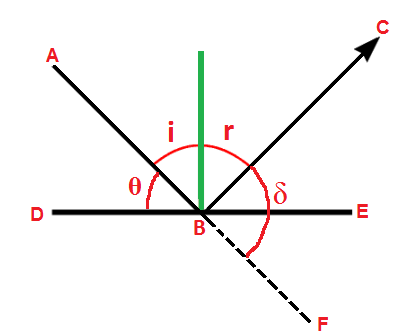Physics

# Reflection at Plane and Curved Surfaces

Optics is the branch of physics which deals about the phenomenon of light.

Real image: An image is said to be real, when the rays of light producing the image actually pass through the position of image.

Virtual image: An image is said to be virtual, when the rays of light producing the image do not actually pass through the position of image but only appear to come from that point.Spherical mirror: A mirror which forms a part of a spherical reflecting surface is called the spherical mirror or curved mirror.Parabolic mirror: A mirror which has reflecting surface in the shape of parabola is called the parabolic mirror.

Magnification: It is as the ratio of the size of image formed by the spherical mirror to the size of the object.

Focal length of a mirror: The distance between the pole and principle focus of a mirror is called the focal length.

1. Focal length of a mirror does not depend on refractive index of the surrounding medium.
2. Image formed by a plane mirror is always virtual, erect and laterally inverted.
3. The field of view of the convex mirror is maximum and least that of concave mirror.
4. For all the colour of light the mirror has same focal length.
5. Regular reflection produces image and irregular does not.
6. There is only one defect of the spherical mirror called spherical abberation.
7. The virtual image formed by a concave mirror is larger than that of the object but in the convex mirror the image is smaller than that of the object.
8. Minimum height of the mirror required is half the height of the man to observe the full image of the man.
9. If the mirror is rotated by an angle then the reflected ray rotates by 2.

Deviation of light by plane mirror

Glancing angle is the angle made by an incident ray with the reflecting surface. It's value in a mirror varies from 0at grazing incidence to 90at the normal incidence.The angle of deviation of a ray by a plane mirror is equal to the twice of the glancing angle. i.e. =2

Law of Rotation of light :When a mirror rotates through an angle , then the reflected ray rotates through an angle 2.

• f=
• =+
• m=====
• Newton's formula, f=, where x1 and x2 are the distances of the object and real image from the focus.
• Number of images produced by two inclined mirror = -1